# Texas Go Math Grade 4 Lesson 18.5 Answer Key Financial institutions

Refer to our Texas Go Math Grade 4 Answer Key Pdf to score good marks in the exams. Test yourself by practising the problems from Texas Go Math Grade 4 Lesson 18.5 Answer Key Financial institutions.

## Texas Go Math Grade 4 Lesson 18.5 Answer Key Financial institutions

Essential Question

What are the purposes of financial institutions?

Financial institutions, such as banks and credit card companies, are businesses.
They are in the business of keeping money safe, lending money, and borrowing money.

When you open a savings account at a bank, the bank is a borrower. This has the advantage of keeping your money safe. When you take out a loan or credit card, the bank is a lender.

Unlock the Problem

Read each situation. Is the financial institution acting as lender or as borrower? Write the letters for each below.

Lending and Borrowing

Financial Institution as Lender: when you take a loan

Financial Institution as Borrower: when you open a savings account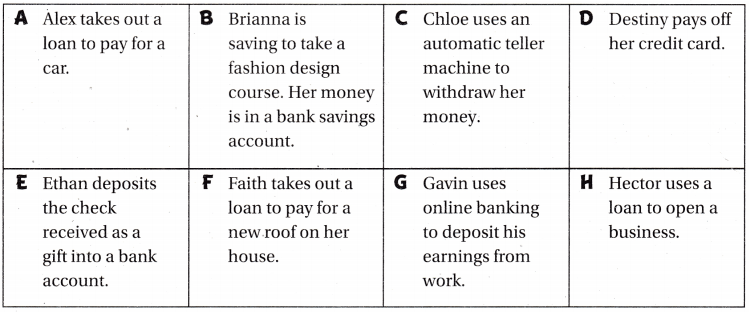Circle the situations above where money is being kept safe.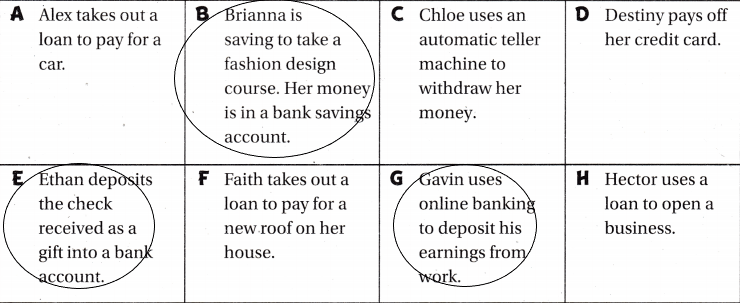When you borrow money from a financial institution, you will pay interest on the loan. Interest is the additional money paid by a borrower to a lender in exchange for the use of the lender’s money.

Example Interest

Tyler wants to buy a 4-wheeler that costs $400. Tyler doesn’t have that much money. He can borrow the money from a financial institution to buy the 4-wheeler, but he will have to pay interest. How much will Tyler have to repay the bank to borrow the money?Find the total interest on$400.

Think: How many $100 are in$400?
Interest = $________ for every$100 borrowed × 4 = $__________ Think: Repayment of loan = loan amount + interest Total amount paid to bank:$__________ + $__________ =$__________
Interest=$10 for every$100 borrowed×4=$40 Total amount paid to bank:$400+$40=$440.

Share and Show

Question 1.
Zack gets a loan for $500 from the bank. The interest rate for the loan is$11 for every $100 borrowed. How much will Zach have to repay the bank to borrow the money? Interest =$ __________ for every $100 borrowed × __________ =$ __________
Total amount paid to hank: $__________ +$ __________ = $__________ Answer: The amount Zack gets a loan from the bank=$500
The rate of interest for every $100=$11
We need to calculate the amount Zack have to repay the bank to borrow the money.
Interest=$11 for every$100 borrowed ×5=$55. Think: Repayment of loan = loan amount + interest Total amount paid to bank:$500+$55=$555.

Write borrower or lender.

Question 2.
When you take out a loan from a bank, you are the __________ and the bank is the __________ .Explanation:
The bank is giving the money to the person that’s why the bank is the lender and those who are taking the money is the borrower.

Question 3.
When you put money into a savings account at a bank, you are the __________ and the bank is the __________ .Explanation:
Here the person is depositing the money in his savings account at a bank means you are saving them and also you are giving money to the bank that’s why you are the lender and the bank is taking so it is a borrower.

Question 4.
Circle the situations where you might use a financial institution.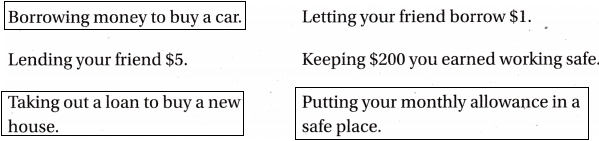Explanation:
I can use financial institutions by taking loans and keeping money safe means depositing money into my savings account at a bank.
If we want to buy a car, bike, house etc. we can borrow money from the bank as a loan.

Problem Solving

Question 5.
Apply Mrs Artino takes out a loan from a financial institution. The loan is for $600. The total interest paid will be$6. How much is the interest per $100 borrowed? Answer:$636.
Explanation:
The amount Artino gets a loan from the bank=$600 The rate of interest for every$100=$6 We need to calculate the amount Artino have to repay the bank to borrow the money. Interest=$6 for every $100 borrowed ×6=$36.
Think: Repayment of loan = loan amount + interest
The total amount paid to bank:$500+$36=$636. Question 6. Write Math when might it be better to use a bank account to save$200 by paying $20 a month than to borrow$200 using a bank loan? Use an example to explain.
In my point of view, saving $20 a month is the best option because I don’t want to pay any interest. But when I take money from the bank as a loan definitely I have to pay interest. Sometimes we need an urgent requirement of money then we have to take the loan because there is no other option than that. For suppose, I want to pay the college fee next year, saving the money before a year is a good option than taking a loan from the bank. For example, I have to pay the college fee of$500 in 6 months then I have to save money 6 months before onwards. If I plan like that, I don’t pay any interest. If I take a loan of $500 with an interest$5 for every $100 then every month I have to pay$525.
Another example: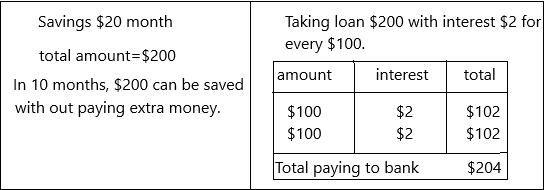Question 7.H.O.T. Multi-Step Sonia takes out a loan for $700 to buy a new washing machine. The interest is$8 for every $100 borrowed. If she wants to repay the loan in 6 months, how much will she need to pay each month? Answer: She has to pay$126 each month.
Explanation:
The amount Sonia gets a loan from the bank=$700 The rate of interest for every$100=$8 We need to calculate the amount Sonia have to repay the bank within 6 months so the amount she needs to pay monthly be ‘X’. Interest=$8 for every $100 borrowed ×7=$56.
Think: Repayment of loan = loan amount + interest
The total amount paid to the bank:$700+$56=$756. X=756/6 X=126 each day. Question 8. H.O.T. Look at the interest paid column in the table. Suppose you borrow$600. Complete the table. Which loan would you choose? Explain your answer.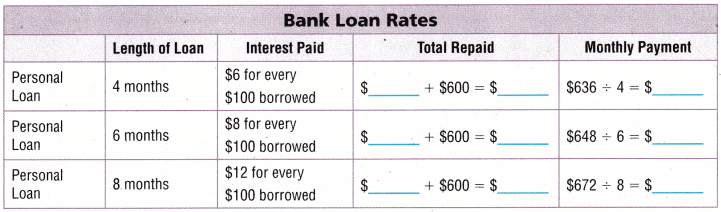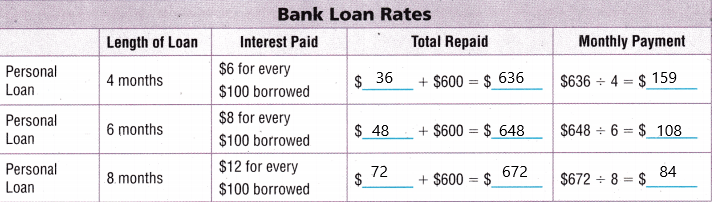Explanation:
I will choose 4 months loan because the interest rate is low. The total repaid amount becomes low.

Question 9.
Which situation shows a financial institution as a borrower?
(A) Abby uses a credit card to pay for an online purchase.
(B) Kevin borrows money to pay for a course as a dental assistant.
(C) Luis deposits his paycheck into a savings account.
(D) Hailey takes out a loan to help her buy a house.Explanation:
Luis is depositing the money means he is giving money to the bank and also saving his money. So that he can earn interest from the bank.

Question 10.
Sean borrows $300 from his bank. The interest is$7 for every $100 borrowed. How much will Sean be charged in interest? (A)$21
(B) $7 (C)$307
(D) $30 Answer: Option A is correct.Explanation: The amount Sean borrows from the bank is$300.
Interest=$7 for every$100 borrowed ×3=$21. Question 11. Multi-Step Casey borrows$200 from her bank. What is the total amount she will have to repay the bank?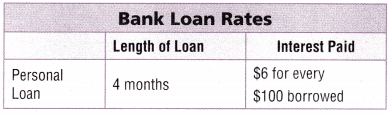(A) $120 (B)$50
(C) $12 (D)$212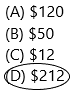Explanation:
Explanation:
The amount Casio gets a loan from the bank=$600 The rate of interest for every$100=$6 We need to calculate the amount Casio have to repay the bank to borrow the money. Interest=$6 for every $100 borrowed ×2=$12.
Think: Repayment of loan = loan amount + interest
The total amount paid to the bank:$200+$12=$212. TEXAS Test Prep Question 12. Which statement is NOT true about a financial institution? (A) A financial institution is a safe place to keep your money. (B) A financial institution charges a fee, called interest, on loans. (C) A financial institution can issue credit cards. (D) A financial institution is a business that never borrows money. Answer: Option D is correct. Financial institution borrows money which is nothing but a person depositing money in his/her savings account.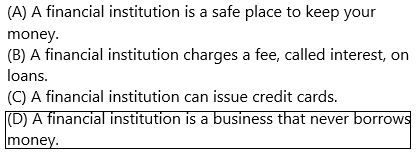### Texas Go Math Grade 4 Lesson 18.5 Homework and Practice Answer Key Question 1. Alexia charges$700 on her credit card. The interest on the credit card is $18 for every$100 borrowed. How much will Alexia have to pay back?
Interest = $_________ per$100 borrowed × __________ = $__________ Total amount paid back =$ _________ + $_________ =$ ___________
Answer: $826 Explanation: The amount Alexia charges on her credit card=$700
The rate of interest for every $100=$18
We need to calculate the amount Zack have to repay.
Interest=$18 for every$100 borrowed ×7=$126. Think: Repayment of loan = loan amount + interest Total amount paid to bank:$700+$126=$826.

Write borrower or lender.

Question 2.
Lucas charges $128 on his credit card. Answer: Lucas is the borrower. The credit card is the lender. Lucas has taken money from the credit card and he is charged$128.
Question 3.
Sophia puts S20 a week into her bank savings account.
Sophia is a lender
The bank is a borrower.
Sophia is depositing the money in her savings account at a bank.

Question 4.
Lillian has some of her paychecks automatically deposited into her savings account.
Lillian is a lender.
The bank is a borrower.
Lillian is depositing the money in her savings account at a bank through paychecks.

Question 5.
Levi gets a loan from the bank to buy a house.
Levi is a borrower.
The bank is a lender.
Levi is taking a loan from the bank to buy a house.

Problem Solving

Question 6.
Mathew borrows $8,200 from the bank to buy a car. The interest on the loan is$6 for every $100 borrowed. How much will Mathew pay back? Answer: Explanation: The amount Mathew borrows from the bank=$8200
The rate of interest for every $100=$6
We need to calculate the amount Mathew have to repay.
Interest=$6 for every$100 borrowed ×82=$492. Think: Repayment of loan = loan amount + interest The total amount paid to the bank:$8200+$492=$8692.

Question 7.
Ellie wants to buy a computer for $900. She can save$75 a month in a savings account and earn $2 for every$100 saved and buy the computer when she has enough money. Or, she can pay back $75 a month on a bank loan for$900 and pay interest of $7 for every$100 borrowed. How much will she pay for the computer for each of the 2 choices?
If she saves money every month:
The amount Ellie wants to buy a computer=$900 The amount she can save each month in her savings account is$75
The amount she can earn for every $100=$2.
The number of months she needs to save $75 to buy a computer=900/75=12 She can earn interest for every 3 months at the time of taking the amount in her account was$918.
If she takes a loan with $7 interest: The amount Ellie borrows from the bank=$900
The rate of interest for every $100=$7
We need to calculate the amount Ellie have to repay.
Interest=$7 for every$100 borrowed ×9=$63. Think: Repayment of loan = loan amount + interest The total amount paid to the bank:$900+$63=$963.
I think she saves $75 every month and then she will pay for the computer because if she saves in her account she gets extra money. If she takes loan then she has to pay an extra amount. Question 8. Why might the Elbe save for the computer? Why might she borrow money to buy the computer? What are the advantages of each way? Answer: I think she saves$75 every month and then she will pay for the computer because if she saves in her account she gets extra money. If she takes a loan then she has to pay an extra amount.
1. Cost-effective: One of the major advantages of taking a bank loan is that it is cost-effective in terms of interest rates. In comparison to other types of loans such as credits cards and overdrafts, a bank loan comes with reasonable interest rates. As such, it will be much easier (and faster) for a company to settle its ‘debt’ when it takes out a bank loan.
2. Retained profits: A bank loan ensures that a business retains all of its profits. When we juxtaposition a bank loan and equity, one notes that with equity, a company surrenders part of its shares to shareholders who in turn will benefit from the company’s profits. This is not the case however with a bank loan as all that the company has to part with is the principal and the interest amount, all profits solely remain with the company.
1. Your money is kept safe: Because your money is being held by a third party, it increases your personal safety. Not only does storing cash on your property make you a target for a potential robbery, but losses like that are not always covered by a homeowner’s or renter’s insurance policy. If there was a fire in your home or some other natural disaster, you could lose your cash as well. Keeping your cash in a savings account keeps you and your money safer.

Lesson Check

Question 9.
Which situation shows a financial institution as borrower?
(B) a shopper uses a credit card to buy a coat
(C) a student borrows money to go to college
(D) a customer makes a deposit into a savings account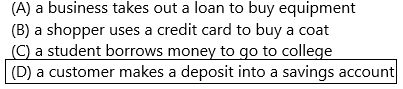Question 10.
Emma borrows $1,300 from her bank. The interest fees are$5 for every $100 borrowed. How much will (A)$75
(B) $130 (C)$65
(D) $50 Answer: Option C is correct.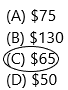Explanation: The amount Emma borrows from the bank is$1300.
Interest=$5 for every$100 borrowed ×13=$65. Question 11. Which statement describes what a financial institution does with the money a customer deposits into a savings account? (A) It makes loans to other customers. (B) It donates the money to charity. (C) It pays the employees of the bank. (D) It pays back loans made to other customers. Answer: Option A is correct.Question 12. Freddie borrowed$600 from a bank to buy a new table saw. The interest payment wall be $30. What is the interest for each$100 borrowed?
(A) $3 (B)$6
(C) $5 (D)$18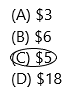Explanation:
The amount Freddie borrows from the bank=$600 The rate of interest for every$100=$X The interest payment =$30
We need to calculate the interest
At what interest, $30 will be the answer. If I keep$5 then I get $30. Interest:$5 for every $100 borrowed ×6=$30

.Question 13.
Multi-Step Sophie borrows $500 for 4 months. She will pay$4 interest for every $100 she borrows. What are her monthly payments? (A)$125
(B) $130 (C)$129
(D) $520 Answer: Option B is correct.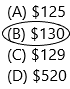The amount Sophie borrows for 4 months=$500
The interest she pays for every $100=$4
Interest=$4 for every$100 borrowed ×5=$20 Think: Repayment of loan = loan amount + interest The total amount paid to the bank:$500+$20=$520.
The monthly payments are 520/4=$130. Question 14. Multi-Step Roger charges$800 on his credit card. He is charged interest of $16 for every$100 borrowed. How much will Roger pay back?
(A) $928 (B)$960
(C) $128 (D)$816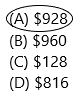The amount Roger charges on his credit card=$800 The rate of interest for every$100=$16 We need to calculate the amount Roger have to repay. Interest=$16 for every $100 borrowed ×8=$128
The total amount paid to the bank:$800+$128=\$928.